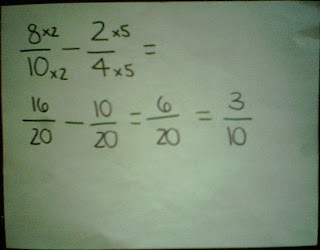### Arielle's Last Fraction Post

Monday, May 11, 2009Find the LOWEST COMMON DENOMINATOR
 Add up the numerators with the new denominator
 Simplify fraction

 Convert the mixed numbers into IMPROPER FRACTIONS
 Find the lowest common denominator
 Add up the numerators with the new denominator
 Convert the fraction to a mixed number
 Simplify fraction

Subtracting Fractions

 Find the lowest common denominator
 Subtract the numerators with the new denominator
 Simplify fractionMultiplying Fractions

 Multiply the numerators together
 Multiply the denominators together
 Simplify fraction

Multiplying Mixed Numbers
 Convert the mixed numbers into improper fractions
 Multiply the numerators together
 Multiply the denominators together
 Convert the fraction to a mixed number
 Simplify fraction

Dividing Fractions
 Turn the second fraction into a reciprocal (upside down)
 Multiply the first fraction to the second fraction
 Convert the fraction to a mixed number
 Simplify fraction

Dividing Mixed Numbers
 Convert the mixed numbers into improper fractions
 Find the reciprocal of the second fraction
 Multiply the first fraction to the second fraction
 Convert the fraction to a mixed number
 Simplify fraction

Problem Two
It takes 2 1/4 scoops of flour to make one cake. How many cakes do 15 scoops of flour make?Problem Three

One day in WInnipeg with 10 1/2 hours of daylight, it was sunny for 1/3 of that time. For how many hours was it sunny that day?